Application of Phase Rule to One Component System

# Application of Phase Rule to One Component System Notes | Study Physical Chemistry - Chemistry

## Document Description: Application of Phase Rule to One Component System for Chemistry 2022 is part of Phase Equilibrium for Physical Chemistry preparation. The notes and questions for Application of Phase Rule to One Component System have been prepared according to the Chemistry exam syllabus. Information about Application of Phase Rule to One Component System covers topics like and Application of Phase Rule to One Component System Example, for Chemistry 2022 Exam. Find important definitions, questions, notes, meanings, examples, exercises and tests below for Application of Phase Rule to One Component System.

Introduction of Application of Phase Rule to One Component System in English is available as part of our Physical Chemistry for Chemistry & Application of Phase Rule to One Component System in Hindi for Physical Chemistry course. Download more important topics related with Phase Equilibrium, notes, lectures and mock test series for Chemistry Exam by signing up for free. Chemistry: Application of Phase Rule to One Component System Notes | Study Physical Chemistry - Chemistry
 1 Crore+ students have signed up on EduRev. Have you?

7. Pure substance

For pure substances C = 1 so that F = 3 − P. In a single phase (P = 1) condition of a pure component system, two variables (F = 2), such as temperature and pressure, can be chosen independently to be any pair of values consistent with the phase. However, if the temperature and pressure combination ranges to a point where the pure component undergoes a separation into two phases (P = 2), F decreases from 2 to 1. When the system enters the two-phase region, it becomes no longer possible to independently control temperature and pressure.

In the phase diagram to the below, the boundary curve between the liquid and gas regions maps the constraint between temperature and pressure when the single-component system has separated into liquid and gas phases at equilibrium. The only way to increase the pressure on the two phase line is by increasing the temperature. If the temperature is decreased by cooling, some of the gas condenses, decreasing the pressure. Throughout both processes, the temperature and pressure stay in the relationship shown by this boundary curve unless one phase is entirely consumed by evaporation or condensation, or unless the critical point is reached. As long as there are two phases, there is only one degree of freedom, which corresponds to the position along the phase boundary curve.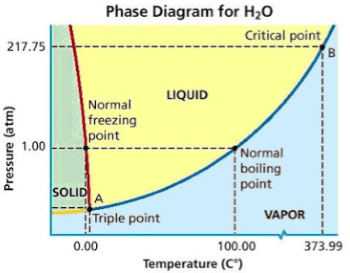The critical point is the black dot at the end of the liquid–gas boundary. As this point is approached, the liquid and gas phases become progressively more similar until, at the critical point, there is no longer a separation into two phases. Above the critical point and away from the phase boundary curve, F = 2 and the temperature and pressure can be controlled independently. Hence there is only one phase, and it has the physical properties of a dense gas, but is also referred to as a supercritical fluid. Of the other two-boundary curves, one is the solid–liquid boundary or melting point curve which indicates the conditions for equilibrium between these two phases, and the other at lower temperature and pressure is the solid–gas boundary.

Even for a pure substance, it is possible that three phases, such as solid, liquid and vapour, can exist together in equilibrium (P = 3). If there is only one component, there are no degrees of freedom (F = 0) when there are three phases. Therefore, in a single-component system, this three-phase mixture can only exist at a single temperature and pressure, which is known as a triple point. Here there are two equations μsol(T, p) = μliq(T, p) = μvap(T, p), which are sufficient to determine the two variables T and p. In the diagram for CO2 the triple point is the point at which the solid, liquid and gas phases come together, at 5.2 bar and 217 K. It is also possible for other sets of phases to form a triple point, for example in the water system there is a triple point where ice I, ice III and liquid can coexist.

If four phases of a pure substance were in equilibrium (P = 4), the phase rule would give F = −1, which is meaningless, since there cannot be −1 independent variables. This explains the fact that four phases of a pure substance (such as ice I, ice III, liquid water and water vapour) are not found in equilibrium at any temperature and pressure. In terms of chemical potentials there are now three equations, which cannot in general be satisfied by any values of the two variables T and p, although in principle they might be solved in a special case where one equation is mathematically dependent on the other two. In practice, however, the coexistence of more phases than allowed by the phase rule normally means that the phases are not all in true equilibrium.

8.  One component system

Examples of one component system are water system, sulphur system, carbon dioxide system, carbon system, phosphorus system etc. Here we will discuss only first three systems etc. Hence we will discuss only first three systems.

In one component system C = 1, so phase rule

F = C – P + 2 reduces to F = 1 – P + 2 or F = 3 – P

Minimum number of phases in a system is 1(P = 1) so maximum number of degrees of freedom is 2(F = 2) for  a one component system. Thus maximum number of variables which are required to express the system are two and those are pressure and temperature. Since minimum number of degree of freedom may be 0(F = 0), so maximum number of phases in equilibrium are 3(P = 3) Two important conclusions may be drawn from above facts:

(i) One component system may be expressed by two variables i.e., phase diagram can be expressed by two six pressure and temperature. This diagram is called temperature pressure diagram.

(ii) Maximum three phase can remain in equilibrium. If one is carried out at a constant temperature and pressure it is required to add component system.

(A) Water System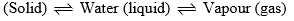Detailed Phase diagram of water system is shown in figure which consists of points of intersection of curves and area surrounded by curves.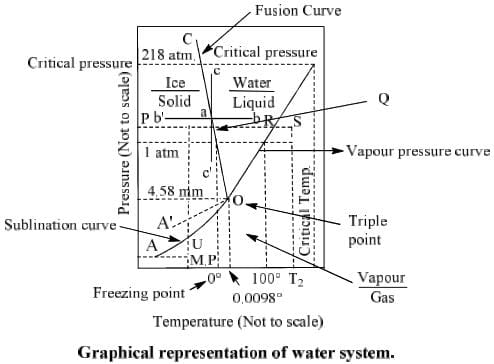(i) Curve

(a) Curve OA: This curve shows solid-vapour equilibrium. It is also called as sublimation curve. Following equilibrium establishes on this curve.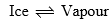There are two phases along this curve (P = 2) and number of component is one (C = 1). Therefore

F = C – P + 2  or    F = 1 – 2 + 2 = 1

Thus degree of freedom along this curve is one, so we require only one variable either temperature or pressure to express the system. Other variable will automatically be fixed. Lower end of curve A goes upto absolute temperature below which temperature cannot be lowered.

(b) Curve OB:   This curve is known as the vapour pressure curve because it gives the vapour pressure of water at different temperature. Following equilibrium establishes along this curve.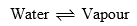The curve starts from O which is the freezing point of water and ends at B, the critical temperature of water (374°C). Beyond this point B the two phases liquid water and vapour merge into each other. There are two phases along this curve (P = 2) and C = 1, therefore system is monovarient (F = 1) along this curve. From this curve it is clear that for any given temperature, there exists a fixed value of pressure. The pressure at critical temperature (374°C) is 218 atm.

(c) Curve OC:  It is the melting point or fusion curve of ice as it indicates the effect of pressure on melting point of ice. Following equilibrium establishes along this curve.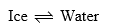The inclination of curve OC along y axis indicates that the melting point of is lowered by increase of pressure. At any point on the curve OC two phases ice and liquid water are in equilibrium (P = 2). It is also monovarient system (F = 1). It means that for any given pressure, melting point must have one definite value.

(d) CurveOA':

It is called super cooling curve. Hence water vapour are in the two phases but water is in metastable state.

(ii) Area enclosed by curves

Whole area is divided into three parts by different curves.

(a) Area surrounded by curves. OA, OC and pressure axis (area AOC). There is only one phase (solidice) in this area. This P = 1, C = 1.

From phase rule

F = C – P + 2

F = 1 – 1 + 2 = 2

Degree of freedom is 2. Therefore we require two variable i.e. pressure and temperature to express the system. It is clear from the fig. that two coordinates are required to locate any point. Therefore it is a bivariant system.

(b) Areas surrounded by curve OA, OB and temperature axis (Area AOB): In this area only vapour exists (P = 1). Hence also degree of freedom is 2 since it is a one component system (C = 1). It is a bivariant system.

(c) Area surrounded by curve OC, OB (area BOC): This area also has only one phase liquid (water). Here also degree of freedom is 2 and system is bivariant.

Significance of Area: Let us take a point ‘a’ on curve OC to understand the significance of areas. At this point two states ice and water, are in equilibrium. Now, if temperature is increased at constant pressure, ice melts into water which is shown by dotted line ab in the fig. Similarly if temperature is decreased as constant pressure, water freezes into ice which is shown by line ab’ in the fig.

Now if pressure is increased at constant temperature ice melts into water and if pressure is decreased, water freezes into ice which are shown by dotted lines ac and ac’ respectively. Similar points canbe taken on curves OA and OB and significance of corresponding areas can be explained.

(ii) Triplet point ‘O’: The three curves OA, OB and OC meet at a point O which is known as triplet point. At this point all the three phases i.e. ice, water and vapour co-exist. Thus the value of P is three. Applying the phase rule to this point.

F = C – P + 2 = 1 – 3 + 2

Or  F = 0

Thus the degree of freedom at triple point is zero. This shows that three phases can co-exist in equilibrium only at a definite temperature and pressure which corresponds to the point. The values of pressure and temperature at this point are 4.50 mm and 0.0098°C. If anyone of these changes one or two phases vanishes.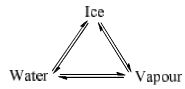Metastable Equilibrium: Sometimes it is possible to cool water below its freezing point without the separation of solid ice. Thus the curve BO can be extended to A’ by cooling below its freezing point without separation of ice. Along the curve OA’ the liquid (water) is in metastable equilibrium with the vapour. Thus the curve OA’ is said to be in metastable equilibrium. As soon as small piece of ice is kept in the contact with the supercooled liquid, it at once changes into the solid ice and curve merges into OA.

From the phase diagram it is seen that the curve OA lies below the curve OA’. Thus the metastable equilibria possess a higher vapour pressure than the stable system if same temperature is considered. Effect of change in temperature and pressure on the system. The real significance of phase diagram can be understood only if we follow the changes that are occurring on changing the pressure or temperature of the system. Suppose we want to see the changes in the system on heating from point P to S at constant pressure or what will be effect of temperature on ice at a certain point V. Since it is a bivariant system, system will shift towards Q on the PO on heating at constant pressure. Ice will be stable from V to Q and starts to melt at 0 and we will get liquid state. Temperature will remains constant until whole ice converts into water and then system starts to move towards R on line OR and temperature will remains constant until whole water changes into vapour. On further increasing the temperature it follows path RS and temperature becomes T2 at S.

Similarly at point T when pressure is decreased at constant temperature, it follows the line UV. From V to U ice is stable and starts to sublime at V and will change into vapour (Univariant system). On further decreasing the pressure, it follows line UW and pressure becomes P2 at W.

Thus behaviour of the system can be studied on varying the temperature and pressure.

Table 1. Important features of water system

 Phase present in system Position in phase diagram Degree of freedom (i) One phase equilibrium water vapour H2O(g) liquid H2O(l) Ice H2O(s) Area below curve AOB Area surrounded by curve OB and OC F = C – P + 2 = 1 – 1 + 2 = 2 (ii) Two phase equilibrium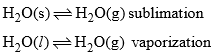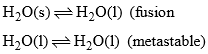Curve AO  Curve OB Curve OC  Curve OA’ F = C – P + 2= 1 – 2 + 2= 1 (iii) Three phase equilibrium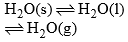Points OTriple point (0.0098°C) F = C – P + 2= 1 – 3 + 2= 0

0°C temperature and 4.58 mm pressure

(B) Sulphur System

It is also a one component system consisting of four phases.

 Rhombic sulphur SR Solid phase Monoclinic sulphur Sm Solid phase Liquid sulphur SL Liquid phase Vapour sulphur SV Vapour phase

This temperature (95.6°C) is called transition temperature. Below this temperature rhombohedral sulphur is stable and above this temperature monoclinic sulphur exists. Melting point of rhombohedral sulphur is 114°C at 0.03 mm. atm pressure whereas monoclinic sulphur melts at 120°C. Liquid sulphur vaporizes at about 450°C. Therefore there are four phases of sulphur which can exist.

If P = 4 is substituted in phase rule F = C – P + 2 for a one component system (C = 1), F comes out to be –1 which is impracticable. Therefore all the four phases cannot coexist together. Maximum three phases can coexist together in sulphur system.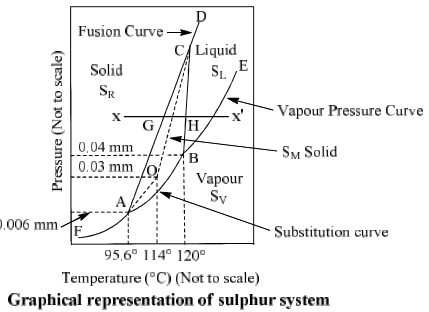The phase-diagram of sulphur system consists of

(i) Curves FA, AB, BE, AC, BC and CD, where two phases are in equilibrium at different temperatures and pressure. They are mono variant.

(ii) Areas: The above curve divide the diagram into four areas where only one phase exists. They are bivariant.

(iii) Triple points: A, B, C and O are four triple points where three phases are in equilibrium at these points. They are nonvariant.

Table: Important characteristics of sulphur system

 Phases present in system one phase equilibrium Position in phase diagram Degree of freedom RhomobohedralSulphur (SR)Monoclinic sulphur (SM) Area left to curve  FACD F = C – P + 2 = 1 – 1 + 2 = 2 Liquid sulphur (SL) Area right to curve EACB Vapour sulphur (SV) Area below to curve FABE

Two phase equilibrium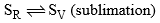Curve FA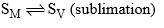Curve AB F = C – P + 2 = 1 – 2 + 2 = 1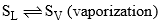Curve BE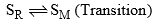Curve AC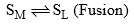Curve BC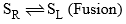Curve CD

Three phase equilibrium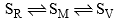Triple point ‘A’ (96.6°C and 0.006 mm Hg) P = C – P + 2 = 1 – 3 + 2 = 0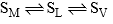Triple point ‘B’ (120°C and 0.06 mm Hg)
The document Application of Phase Rule to One Component System Notes | Study Physical Chemistry - Chemistry is a part of the Chemistry Course Physical Chemistry.
All you need of Chemistry at this link: Chemistry

## Physical Chemistry

67 videos|106 docs|31 tests
 Use Code STAYHOME200 and get INR 200 additional OFF

## Physical Chemistry

67 videos|106 docs|31 tests

Track your progress, build streaks, highlight & save important lessons and more!

,

,

,

,

,

,

,

,

,

,

,

,

,

,

,

,

,

,

,

,

,

;